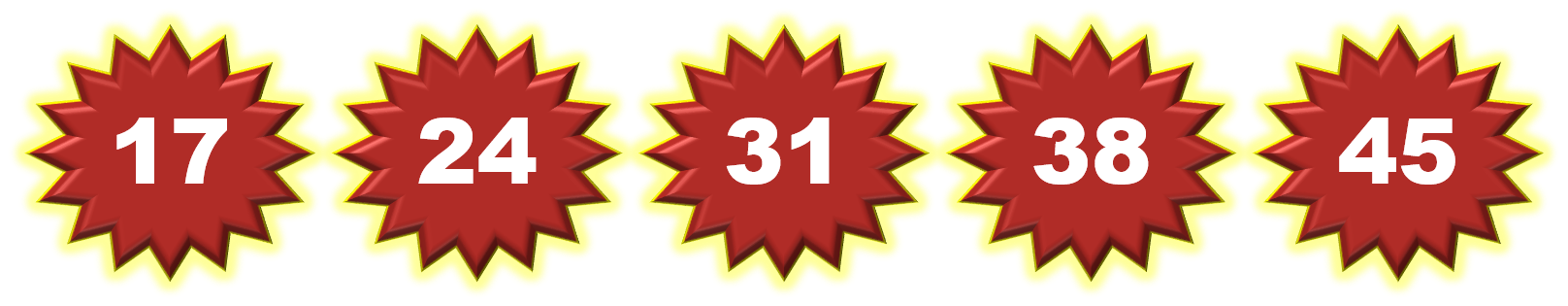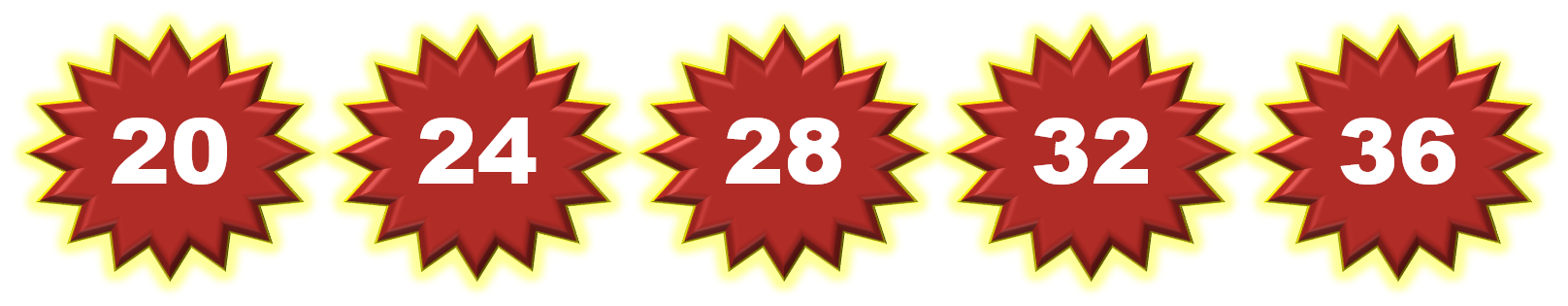1
visibility

Choose the correct counting pattern with the rule ‘add seven’.

• A• BThe correct answer is Option B:Solution:
Considering the given options.

Option A.Option B.On Option B:  Considering the given number pattern.Here, the numbers are increasing.
So, we first find the rule.

Note that:
17 + 7 = 24
24 + 7 = 31
31 + 7 = 38
38 + 7 = 45

So, the rule is "add 7", and this is the required answer.

Hence, the correct counting pattern with the rule ‘add seven’ is Option B: